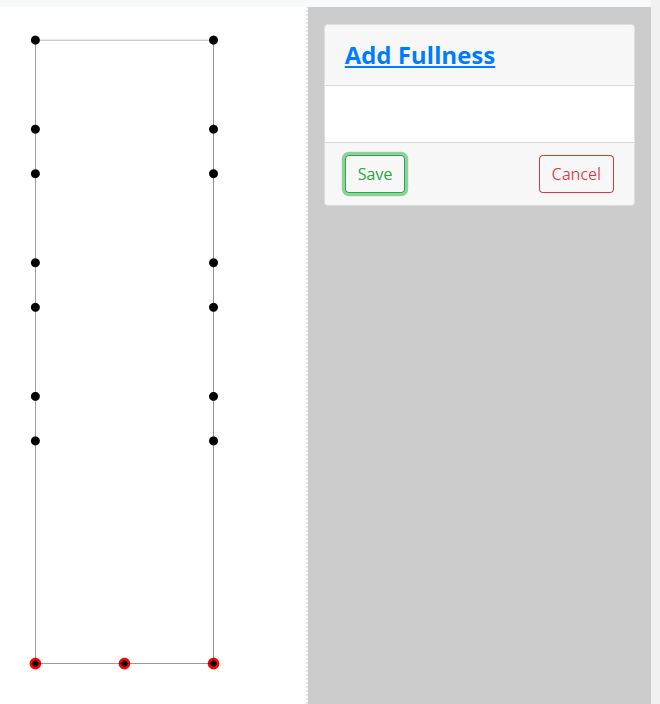This tool adds fullness to the entire pattern. It is a slash and spread technique using two sets of opposing points for slashing. Review the images below for a visual explanation.

## Fullness Balance

You will select two separate sets of points for slashing across the pattern. You have three options in regard to how the sets are spread:

• The same spread is applied to both sets of points.
• One spread is greater on one set of points.
• One set of points has spread, but the other set has none.

Regardless of which method you choose, the first set of points you choose must have the greatest spread value.

## Tool Process

### First Set

Select the first set of points. This set must have the greatest spread value. Always start your point selection at the point furthest from the area that will move.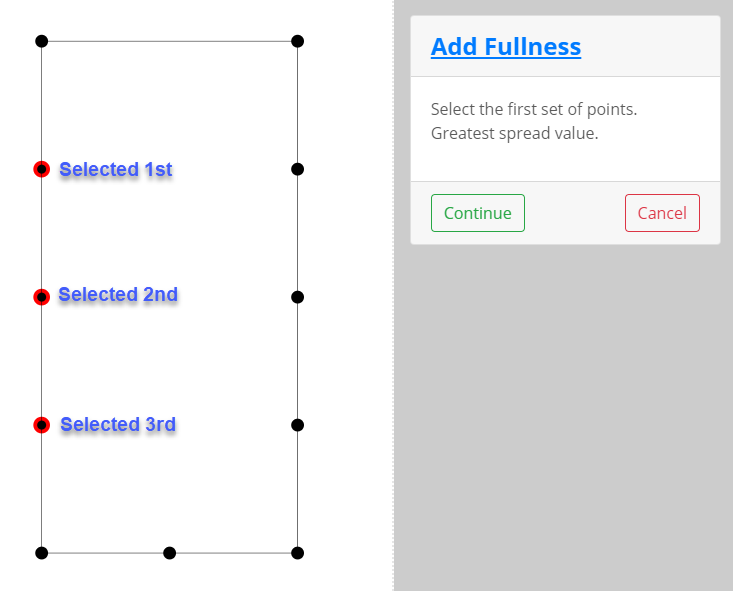### Second Set

Select the opposing set of points. The program will tell you have many points you must select. The first and second sets must have the same number of points.

Also, these points must be selected in the same order (top to bottom or bottom to top) as the first set.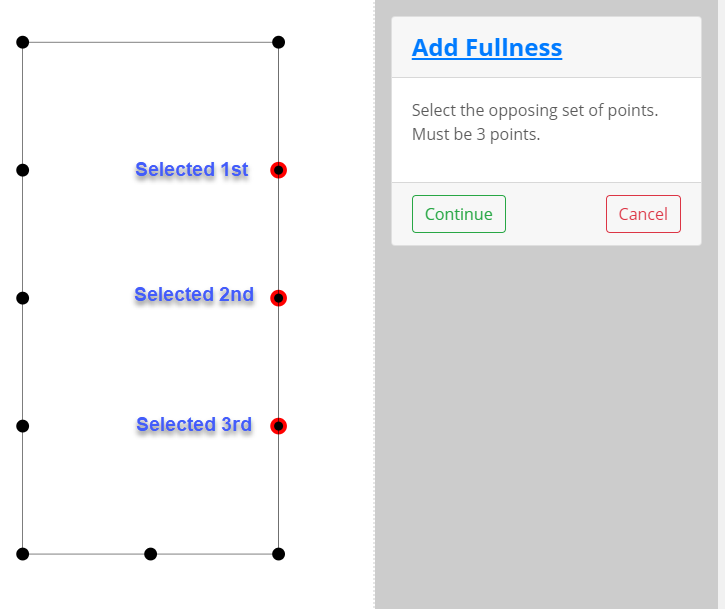### Select Points to Move

In the process of spreading the selected points apart, there are points within the moving "block" that must move. These points are located between the two slash sets. Select those intermediary points.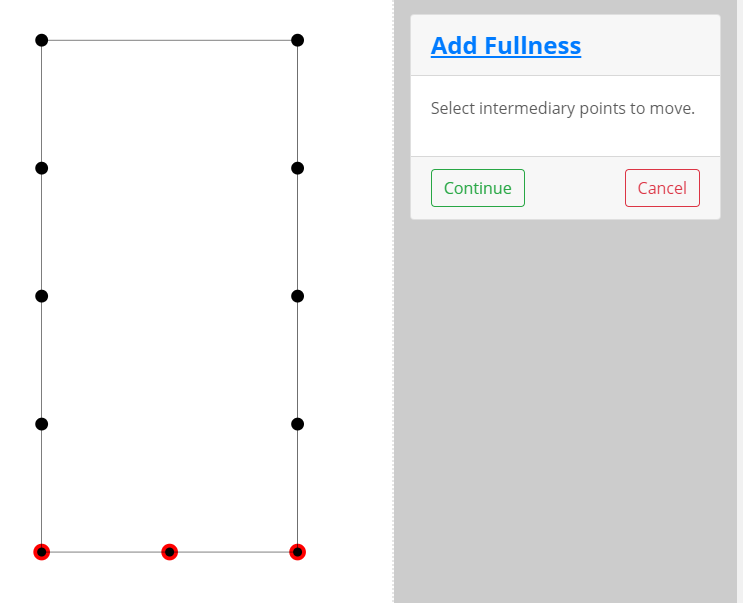Now you can enter the amount of spread that will be added at each slash point. Remember that the total fullness is calculated as:

Number of Slashes X Spread Value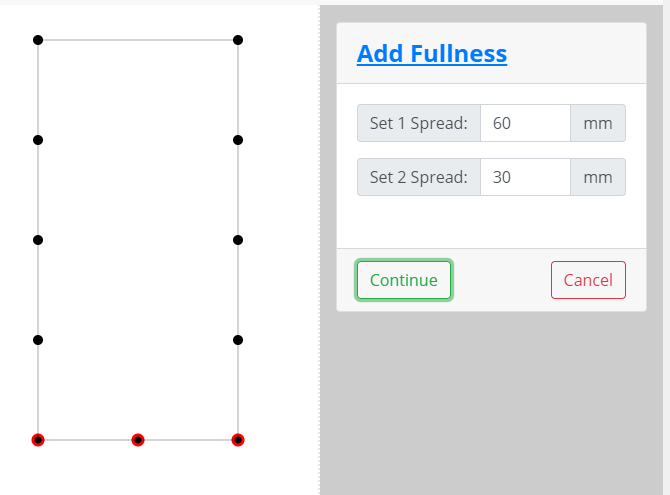## Results

### Unequal Spread - Two Values

This is the result of the settings above. The first set is spread 60mm between slashes and the second set is 40mm. This curves the pattern.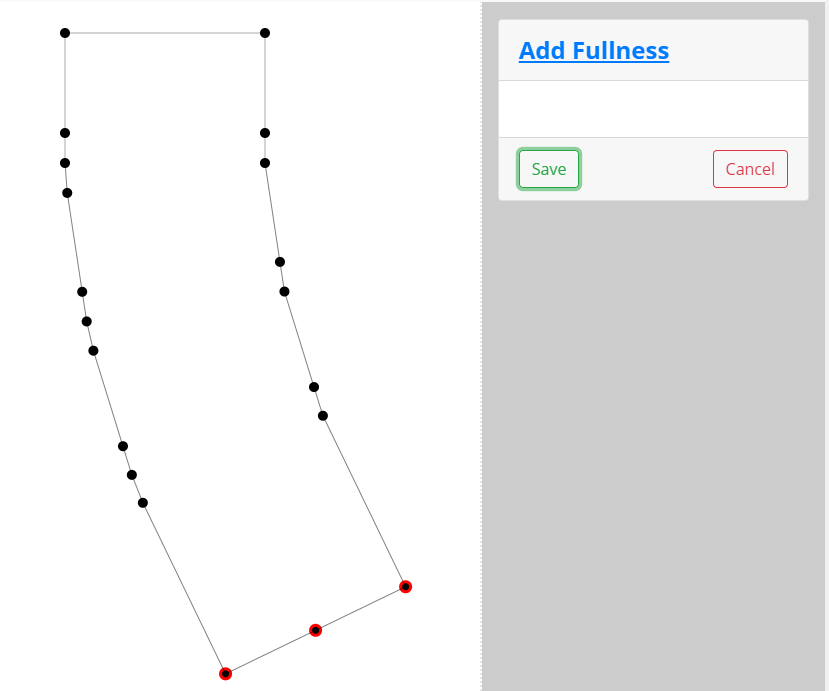### Unequal Spread - One Value

This is the result when a spread value is added to only the first set. Even though the second set didn't spread, it serves as the pivot points for the first set.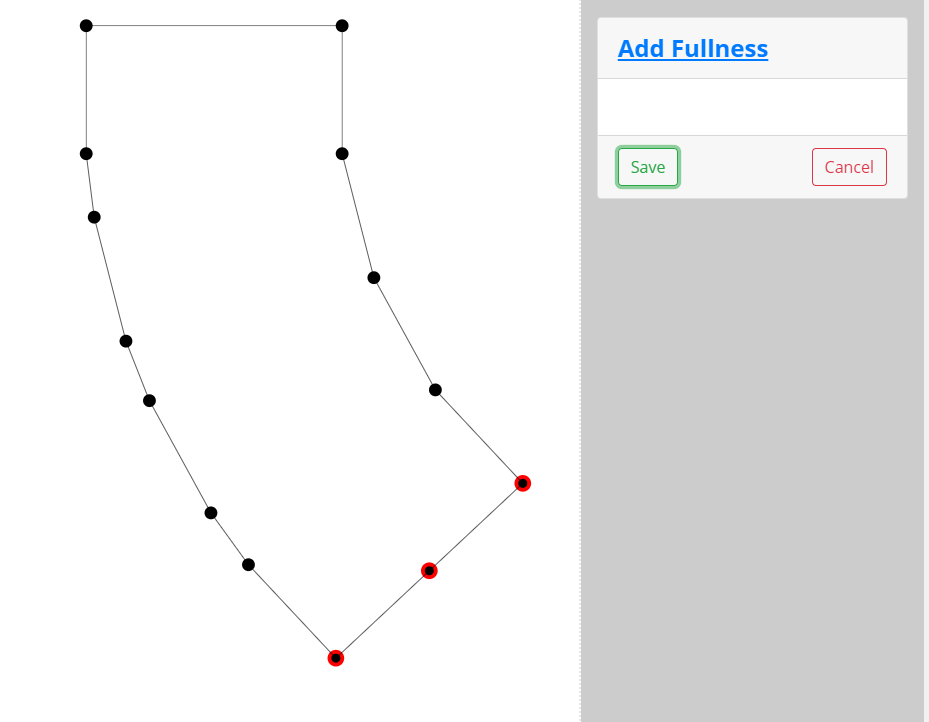### Equal Spread - Two Values

This is the result when the spread value is the same for both sets. The pattern is spread apart equally.